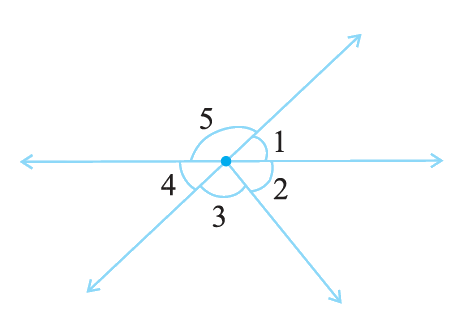# Ex.5.1 Q10 Lines and Angles - NCERT Maths Class 7

Go back to  'Ex.5.1'

## Question

Indicate which pairs of angles are:

(i) Vertically opposite angles?

(ii) Linear pairs?Video Solution
Lines & Angles
Ex 5.1 | Question 10

## Text Solution

Steps:

(i) $$\angle 1$$ and $$\angle 4, \angle 5$$ and ( $$\angle 2+\angle 3$$) are vertically opposite angles as they formed due to intersection of two straight lines.

(ii) $$\angle 1$$ and$$\angle 5 , \angle 5$$ and $$\angle 4$$ forms linear pair.

Learn from the best math teachers and top your exams

• Live one on one classroom and doubt clearing
• Practice worksheets in and after class for conceptual clarity
• Personalized curriculum to keep up with school